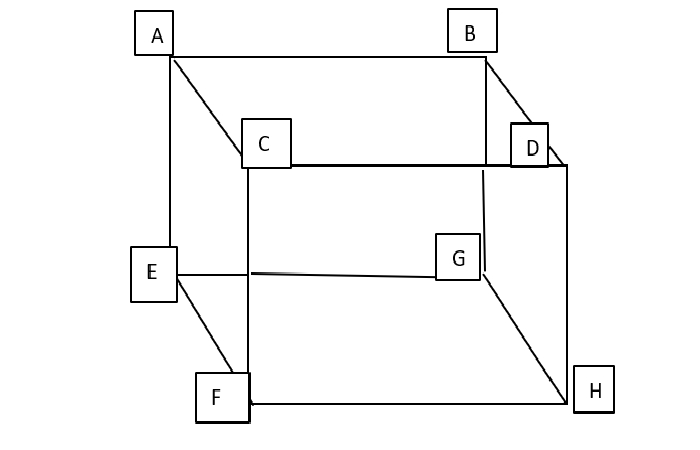Education

# Geometric Shapes: All About Cubes and CuboidsGeometric Shapes: All About Cubes and Cuboids – We are surrounded by geometric shapes in everyday life. These are figures that have a specific boundary and are made up of a definite set of curves, lines, and points. Common geometric shapes that we study in elementary school are squares, triangles, circles, hexagons, pentagons, etc. All these are closed shapes as they are connected at both ends. Three-dimensional shapes are defined by a set of vertices and lines that connect these vertices and enclose two-dimensional faces, for example, cubes and Cuboid. Let us understand this in detail.

## Types of Geometric Shapes

Two-dimensional geometric shapes – These are flat structures having two dimensions and are made up of x-axis and y-axis. For example, square, triangle, rectangle, etc.

Three-Dimensional Geometric Shapes – These are solid structures having three dimensions. They are made up of an x-axis, y-axis, and z-axis that represent the height of an object. Some of the common examples of 3D shapes are cube, cuboid, etc.

## What is a Cuboid?

A cuboid is a 3D geometric shape that is made up of six rectangles. Sometimes, people get confused between a cube and a cuboid as they share the same properties more or less.

## Let us understand the difference between a Cube and a Cuboid?

Both cube and cuboid have six faces, twelve edges, and eight vertices. However, the six faces of a cuboid are rectangles while those of a cube are squares. The surface area, as well as the volume occupied by a cube and a cuboid, is also different. The height, length, and width of a cube are the same whereas they are different in a cuboid.

### Properties of Cuboid1. A cuboid has six faces. If you look at the above figure the six faces of this cuboid are:

ABCD, CDFH, AEBG, EFGH, ACEF, and BDHG.

1. A cuboid has 12 edges which are the sides of the rectangles. These are AB, BD, DC, CA, AE, EF, FC, DH, HF, BG, GE, and GH.
2. A cuboid has eight vertices, A, B, C, D, E, F, G, H.
3. A cuboid has two types of diagonals namely, Space diagonals and Face diagonals. The diagonals connecting the opposite vertices of a face are called face diagonals, therefore a cuboid can have twelve face diagonals. A line segment that joins the opposite vertices of a cuboid is called a space diagonal. Each cuboid can have four space diagonals.
4. The opposite edges of a cuboid are always parallel to each other.
5. All the vertices of a cuboid form a right angle.
6. The total area occupied by a cuboid is known as its surface area. This depends on its length, breadth, and height.
7. The space occupied by a cuboid is called its volume and depends upon the length, breadth, and height. If any one of these is changed, the volume of the cuboid is changed. Volume is measured in (unit)³.

The volume of the cuboid can be calculated by the following formula:

V= lbh

Where,

V= volume

l = length

b = breadth, and

h = height.

## Things to keep in mind while calculating the volume of a cuboid.

Before calculating the volume of a cuboid, make sure that all the given dimensions are in the same unit. If not, convert them to the same unit and then proceed further.

Multiply the values of the length, breadth, and height, and don’t forget to write the unit with the answer.

## Examples of Cuboids in everyday life

The most common example of a cuboid is the books that you read every day. The drawers and cabinets where you keep your books and other things are also mostly constructed in the shape of a cuboid. The mattress that you sleep on, the lunch box that you carry to school, bricks, matchbox are some of the other common examples of a cuboid.

For further clarification, you can take guidance from the experienced teachers associated with Cuemath, a reliable learning platform that has revolutionized the teaching methodology.